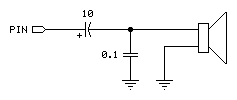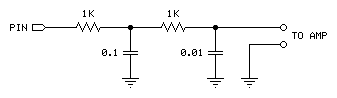SOUND Example

SOUND Pin, Note, Duration

Function
generate square-wave tone for a specified duration.

• Pin  is any SX I/O pin (RA.0 .. RA.7, RB.0 .. RB.7, RC.0 .. RC.7, RD.0 .. RD.7, RE.0 .. RE.7).
• Note  is a byte variable/constant (0 - 127) specifying the frequency of the tone (see calculation below).
• Duration  is a byte variable/constant (1 - 255) specifying the amount of time to generate the tone. The unit of time for Duration is 10 milliseconds.

Explanation
SOUND generates one of 255 square-wave frequencies on an IO pin. The output pin should be connected as shown in the circuits below for audio use.

The tones produced by SOUND can vary in frequency from 94.3 Hz (1) to 11,062 Hz (127). If you need to determine the frequency corresponding to a given Note value, or need to find the note value that will give you best approximation for a given frequency, use the equations below. Note values above 127 are handled as Note - 128, in other words, a Note value of 138 produces the same tone as 10.

 Note INT(127 - (((1 ÷ Frequency) - 0.0000812) ÷ 0.0000805)) Frequency (Hz) 1 ÷ (0.0000812 + ((127 - Note) x 0.0000805))

When driving a Hi-Z speaker (> 40 Ω) or piezo element:When connecting to an audio amplifier:Related instruction: FREQOUT